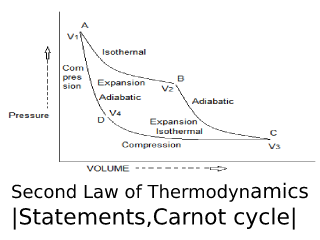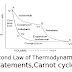## Second Law of Thermodynamics.The various statements of the second law of thermodynamics are:

### Clausius Statement.

"It is impossible to construct a machine which is able to convey heat by a cyclic process from a reservoir at a lower temperature to one at a high temperature unless work is done on the machine by some external agency".
Example.
In a refrigerator, heat from the inner compartment is transferred to the surroundings at a higher temperature at the expenditure of work.

### Kelvin-Planck Statement.

"It is impossible to construct a machine that, operating in a cyclic process, will take heat from a reservoir at constant temperature and convert it into work without accompanying changes in the reservoir or its surroundings".

### Lewis Statement.

"Every system that is left to itself will, on the average, change towards a condition of maximum probability".

"When a system undergoes a reversible change, the total entropy of the system and surroundings remains constant, but when a system undergoes a natural spontaneous change (irreversible) change, the total entropy of the system and surrounding increase".

### The Carnot Cycle.

To calculate the maximum extent to which heat can be converted into work, Nicolas Sadi Carnot, a French chemist devised a hypothetical engine working in a cyclic manner, in four stages. These stages constitute the Carnot cycle. The gas returns to its original state at the end of the fourth step.
In this machine, the working substance is one mole of an ideal gas and it is contained in a cylinder fitted with a frictionless and weightless piston.

It is supposed that two very large reservoirs of heat at T2 and T1 are available. It is also possible to surround the cylinder by a perfect non-conductor, thus, adiabatic processes are possible.

### First Step. (Isothermal Expansion)

The cylinder containing but 1 mole of gas of volume V1 at pressure P1 is placed in the constant temperature reservoir at T2. Let the gas expands isothermally and reversibly to the new volume V2, along AB.

The work W1 done by the gas after taking heat Q2 from the reservoir is given by

At B the gas is at T2 and has a volume V2 under the new pressure P2. It is now completely isolated and is allowed to expand adiabatically along BC to new volume V3.

The work is done at the expense of the kinetic energy of the gas molecules. Therefore, there is a fall of temperature from T2 to T1 and the work done is

W2   = Cv  (T2 - T1)         ......... (ii)

### Third Operation. (Isothermal Compression)

The cylinder is now placed in contact with a hot reservoir at T1 and the gas is compressed isothermally and reversibly from C to D: The final volume is V4, so that the work is done is represented by

Where - Q1 is heat given to the reservoir by gas at T1. In fact, W3 is negative because V3 is greater than V4 and (iii) represents the work done on the gas in compression.

The gas is again isolated and compressed adiabatically along DA until the initial state A is regained. The temperature of the gas rises from T1 to T2 and the volume change from V to V1. The work done upon the gas is

W4   =   - Cv (T2 - T1)          ........... (iv)

The total work is done in this four-stage operation is as:

W   =   W1  +  W2  - W3  + W4

Substituting the values,

Since V1 and V4 lie on one adiabatic curve, and V2 and V3 are on another, it follows that

On substituting these in (v), it is seen that
Dividing (vii) by (i) we get
The factor W/Q2 is called the thermodynamic efficiency of the reversible cycle. It gives the fraction of heat which is possible to convert into work.

The Carnot equation (viii) reveals that the efficiency of a process depends upon the temperature limits between which cycle operates and is independent of the nature of the working substance and other factors.
Second Law of Thermodynamics | Statements, Carnot cycle |Reviewed by Genuine Chemistry on August 19, 2019 Rating: 5